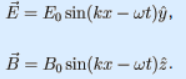# Problem: The simplest form of a traveling electromagnetic wave is a plane wave. For a wave traveling in the x direction whose electric field is in the y direction, the electric and magnetic fields are given by the picture shown above. This wave is linearly polarized in the y direction.1. What is the mathematical expression for the electric field at the point x=0, y= 0, z at time t?2. For a given wave, what are the physical variables to which the wave responds?

###### FREE Expert Solution

1.

The electric field is a function of x and t.

At x = 0,

86% (316 ratings)###### Problem DetailsThe simplest form of a traveling electromagnetic wave is a plane wave. For a wave traveling in the x direction whose electric field is in the y direction, the electric and magnetic fields are given by the picture shown above. This wave is linearly polarized in the y direction.

1. What is the mathematical expression for the electric field at the point x=0, y= 0, z at time t?

2. For a given wave, what are the physical variables to which the wave responds?